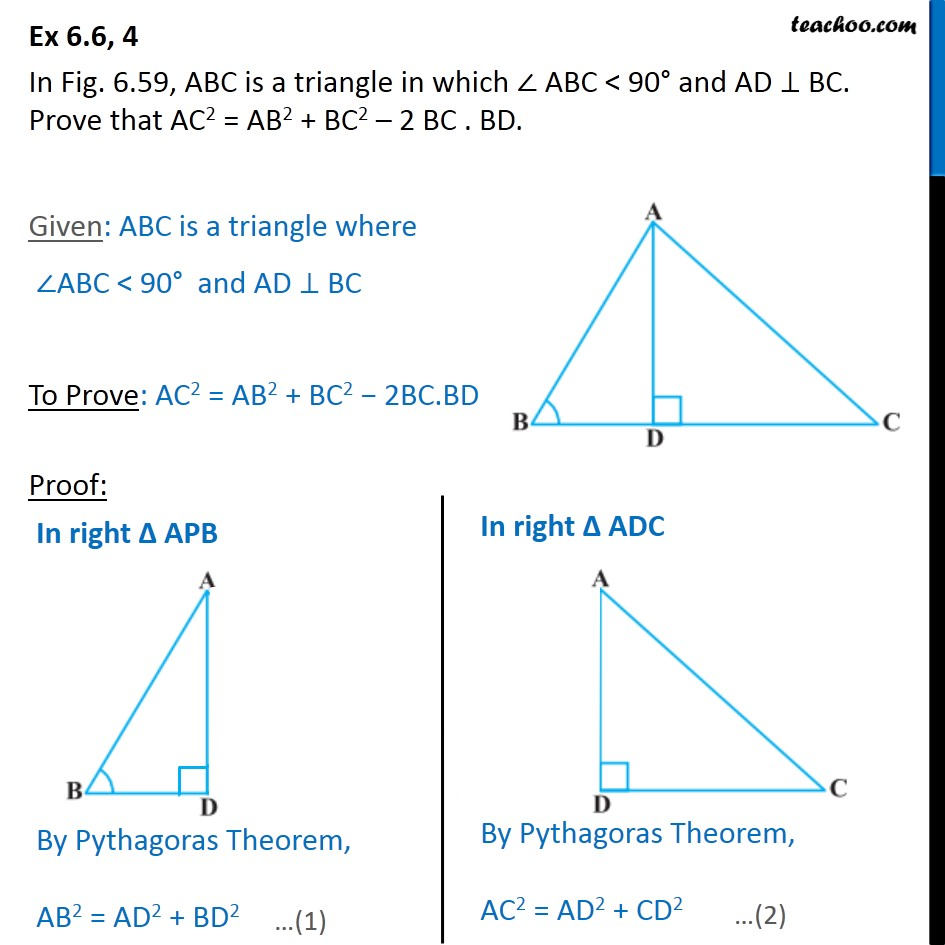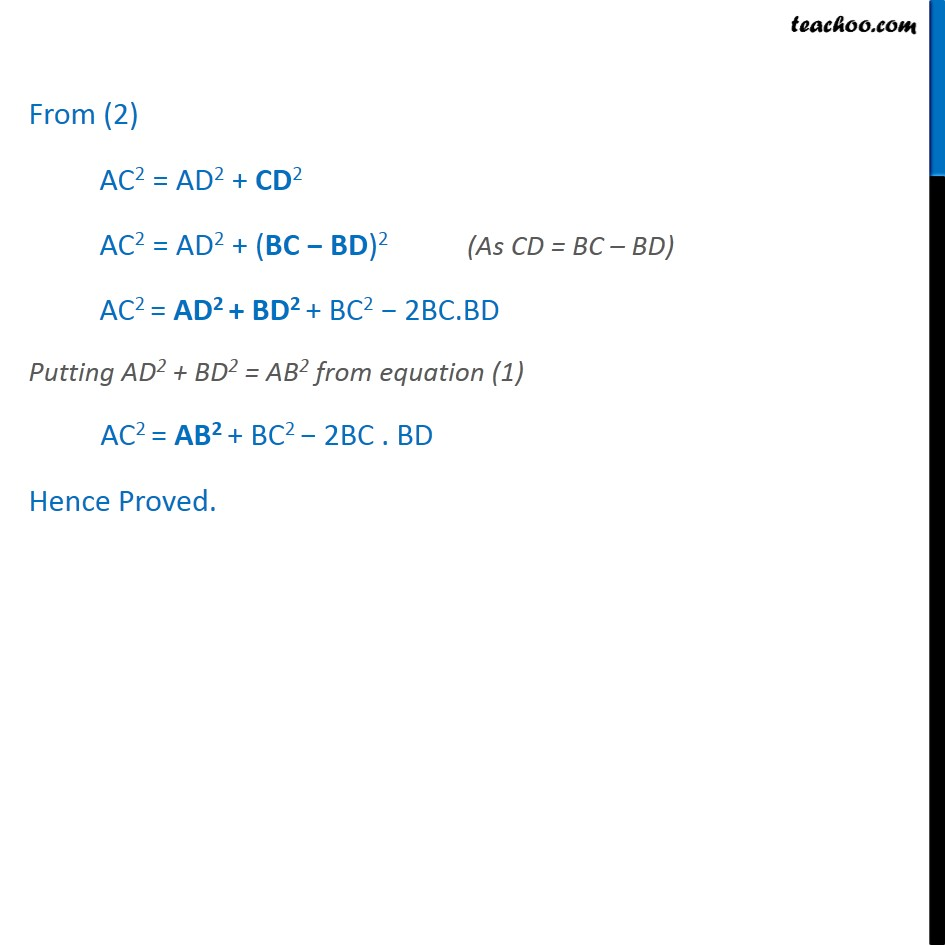Important questions of Triangle (in Geometry)

Chapter 6 Class 10 Triangles
Serial order wiseLearn in your speed, with individual attention - Teachoo Maths 1-on-1 Class

### Transcript

Question 4 In Fig. 6.59, ABC is a triangle in which ABC < 90 and AD BC. Prove that AC2 = AB2 + BC2 2 BC . BD. Given: ABC is a triangle where ABC < 90 and AD BC To Prove: AC2 = AB2 + BC2 2BC.BD Proof: In right APB By Pythagoras Theorem, AB2 = AD2 + BD2 In right ADC By Pythagoras Theorem, AC2 = AD2 + CD2 From (2) AC2 = AD2 + CD2 AC2 = AD2 + (BC BD)2 AC2 = AD2 + BD2 + BC2 2BC.BD Putting AD2 + BD2 = AB2 from equation (1) AC2 = AB2 + BC2 2BC . BD Hence Proved.